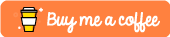# #dotnet – Pose detection from the 🎦 camera feed using #OpenCV and #net5. Home-made #kinect!Hi !

LearnOpenCV is an amazing resource to learn about OpenCV. And, it has lot of scenarios of real life problem solved with OpenCV. Most of the samples are in C++ or Python, so I decided to pick one related to pose estimation, and using .Net 5 in a Winforms App, build something like this:

The main model is OpenPose (see references). The model is amazing, and also works fast: ~1 FPS. There are other variations here, detecting face, Body, Foot, Face, and Hands Estimation, and more. I’ll try and share some of the other models usage in C# in next posts.

Now as usual, a huge code snippet with only the frame recognition and processing to detect the body joints.

```private void CaptureCameraCallback()
{
while (true)
{
if (!_run) continue;
var startTime = DateTime.Now;

if (_image.Empty()) return;
var imageRes = new Mat();
Cv2.Resize(_image, imageRes, new Size(320, 240));
if (_detectPose)
{

var frameWidth = imageRes.Cols;
var frameHeight = imageRes.Rows;

const int inWidth = 368;
const int inHeight = 368;

// Convert Mat to batch of images
using var inpBlob = CvDnn.BlobFromImage(imageRes, 1.0 / 255, new Size(inWidth, inHeight), new Scalar(0, 0, 0), false, false);

_netPose.SetInput(inpBlob);

using var output = _netPose.Forward();
var H = output.Size(2);
var W = output.Size(3);

var points = new List<Point>();

for (var n = 0; n < nPoints; n++)
{
// Probability map of corresponding body's part.
using var probMap = new Mat(H, W, MatType.CV_32F, output.Ptr(0, n));
var p = new Point2f(-1, -1);

Cv2.MinMaxLoc(probMap, out _, out var maxVal, out _, out var maxLoc);

var x = (frameWidth * maxLoc.X) / W;
var y = (frameHeight * maxLoc.Y) / H;

if (maxVal > thresh)
{
p = maxLoc;
p.X *= (float)frameWidth / W;
p.Y *= (float)frameHeight / H;

Cv2.Circle(imageRes, (int)p.X, (int)p.Y, 8, Scalar.Azure, -1);
//Cv2.PutText(imageRes, Cv2.Format(n), new Point((int)p.X, (int)p.Y), HersheyFonts.HersheyComplex, 1, new Scalar(0, 0, 255), 1);
}

}

WriteTextSafe(@\$"Joints {nPoints} found");

var nPairs = 14; //(POSE_PAIRS).Length / POSE_PAIRS.Length;

for (var n = 0; n < nPairs; n++)
{
// lookup 2 connected body/hand parts
var partA = points[posePairs[n]];
var partB = points[posePairs[n]];
if (partA.X <= 0 || partA.Y <= 0 || partB.X <= 0 || partB.Y <= 0)
continue;
Cv2.Line(imageRes, partA, partB, new Scalar(0, 255, 255), 8);
Cv2.Circle(imageRes, partA.X, partA.Y, 8, new Scalar(0, 0, 255), -1);
Cv2.Circle(imageRes, partB.X, partB.Y, 8, new Scalar(0, 0, 255), -1);
}

}
// rest of the code to calc FPS and display the image
}
}
```

Super fun ! and check the references for the model and support files download location.

Happy coding!

Greetings

El Bruno

## References

1.Lai Eddy says:

where can download this “.Net 5 in a Winforms App” .sln /project files?

Like

1.elbruno says:

I don’t know if if I have those files around.
You can create a new Winforms App in NET 6 and use this code !

Like

This site uses Akismet to reduce spam. Learn how your comment data is processed.